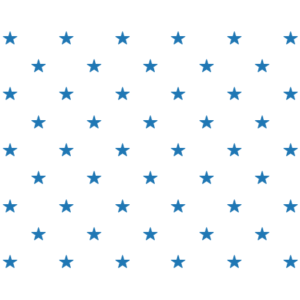## Making something out of nothing

This week’s Fiddler is a problem about composing functions. Here it goes:

Consider $f(n) = 2n+1$ and $g(n) = 4n$. It’s possible to produce different whole numbers by applying combinations of $f$ and $g$ to $0$. How many whole numbers between $1$ and $1024$ (including $1$ and $1024$) can you produce by applying some combination of $f$’s and $g$’s to the number $0$?

Extra Credit: Now consider the functions $g(n) = 4n$ and $h(n) = 1−2n$. How many integers between $-1024$ and $1024$ (including $-1024$ and $1024$) can you produce by applying some combination of $g$’s and $h$’s to the number $0$?

My solution:
[Show Solution]

## Möbius prism

This week’s Fiddler is a problem about a multi-sided Möbius prism:

Consider an three-dimensional prism whose bases are regular N-gons. I twist it and stretch it into a loop, before finally connecting the two bases. Suppose that my twist is by a random angle, such that the two bases are aligned when they are coonected. Among all whole number values of N less than or equal to 1,000, for which value of N will a randomly twisted regular N-gon prism have the most distinct faces, on average?

My solution:
[Show Solution]

## A cube of primes

This week’s Riddler Classic is a question about prime numbers.

Consider a cube, which has eight vertices, or corners. Suppose I assign a prime number to each vertex. A “face sum” is the value I get when I add up all four prime numbers on one of the six faces.

Can you find eight distinct primes and arrange them on a cube so that the six face sums are all equal?

Extra credit: Can you find another set of eight distinct primes that can similarly be arranged on the vertices of a cube? How many more can you find?

Extra Extra credit: Same puzzle for the other four platonic solids.

Here is my solution:
[Show Solution]

## Squaring the pentagon

This week’s Riddler Classic is a number theory problem, which I will paraphrase:

The number 50 can be represented using a set of interleaved dots where the number of columns is one greater than the number of rows; the same way the stars in the US flag are arranged. If we add 1 to this number, we obtain 51, which can be represented using concentric pentagons. Here are diagrams showing both arrangements:What is the next number with the property that it can be represented as interleaved dots but when you add 1 to it it can be represented using concentric pentagons?

Here is my solution:
[Show Solution]

## Penny Pinching

This week’s Riddler Classic is indeed a classic! Here it goes (paraphrased to make it a bit more general):

The game starts with $n$ pennies, which I then divide into two piles any way I like. Then we alternate taking turns, with you first, until someone wins the game. For each turn, a player may take any number of pennies he or she likes from either pile, or instead take the same number of pennies from both piles. Each player must also take at least one penny every turn. The winner of the game is the one who takes the last penny.

If we both play optimally, what starting numbers of pennies guarantee that you can win the game?

Here is my solution:
[Show Solution]

## Prismatic puzzle

This week’s Riddler Classic is simple to state, but tricky to figure out:

What whole number dimensions can rectangular prisms have so that their volume (in cubic units) is the same as their surface area (in square units)?

Here is my solution:
[Show Solution]

## Counting coconuts

This Riddler problem is about divisibility… of coconuts!

Seven pirates wash ashore on a deserted island after their ship sinks. In order to survive, they gather as many coconuts as they can find and throw them into a central pile. As the sun sets, they all go to sleep.

One pirate wakes up in the middle of the night. Being the greedy person he is, this pirate decides to take some coconuts from the pile and hide them for himself. As he approaches the pile, though, he notices a monkey watching him. To keep the monkey quiet, the pirate tosses it one coconut from the pile. He then divides the rest of the pile into seven equally sized bunches and hides one of the bunches in the bushes. Finally, he recombines the remaining coconuts into a single pile and goes back to sleep. (Note that individual coconuts are very hard, and therefore indivisible.)

Later that night, a second pirate wakes up with the same idea. She tosses the monkey one coconut from the central pile, divides the pile into seven bunches, hides her bunch, recombines the rest, and goes back to sleep. After that, a third pirate wakes up and does the same thing. Then a fourth. Then a fifth, and so on until all seven pirates have hidden a share of the coconuts.

In the morning, the pirates look at the remaining central pile and notice that it has gotten quite small. They decide to split the pile into seven equal bunches and take one bunch each. (Note: The monkey does not get one this time.) If there were N coconuts in the pile originally, what is the smallest possible value of N?

Here is a short solution:
[Show Solution]